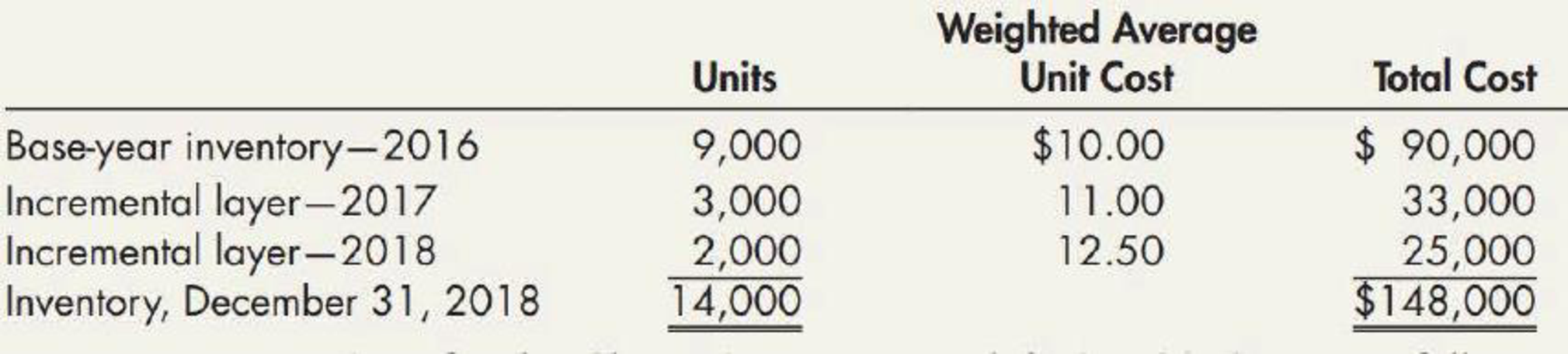Chapter 7, Problem 10P### Intermediate Accounting: Reporting...

3rd Edition
James M. Wahlen + 2 others
ISBN: 9781337788281

#### Solutions

Chapter
Section### Intermediate Accounting: Reporting...

3rd Edition
James M. Wahlen + 2 others
ISBN: 9781337788281
Textbook Problem
12 views

# LIFO and Inventory Pools On January 1, 2016, Grover Company changed its inventory cost flow method to the LIFO cost method from the FIFO cost method for its raw materials inventory. It made the change for both financial statement and income tax reporting purposes. Grover uses the multiple-pools approach under which it groups substantially identical raw materials into LIFO inventory pools. It uses weighted average costs in valuing annual incremental layers. The composition of the December 31, 2018, inventory for the Class F inventory pool is as follows:Inventory transactions for the Class F inventory pool during 2019 were as follows: On March 2, 2019, 4,800 units were purchased at a unit cost of $13.50 for$64,800. On September 1, 2019, 7,200 units were purchased at a unit cost of $14.00 for$100,800. A total of 15,000 units were used for production during 2019. The following transactions for the Class F inventory pool took place during 2020: On January 11, 2020, 7,500 units were purchased at a unit cost of $14.50 for$108,750. On May 14, 2020, 5,500 units were purchased at a unit cost of $15.50 for$85,250. On December 29, 2020, 7,000 units were purchased at a unit cost of $16.00 for$112,000. A total of 16,000 units were used for production during 2020. Required: 1. Prepare a schedule to compute the inventory (units and dollar amounts) of the Class F inventory pool at December 31, 2019. Show supporting computations in good form. 2. Prepare a schedule to compute the cost of Class F raw materials used in production for the year ended December 31, 2019. 3. Prepare a schedule to compute the inventory (units and dollar amounts) of the Class F inventory pool at December 31, 2020. Show supporting computations in good form.

1.

To determine

Prepare a schedule to calculate the inventory in units and dollars of the Class F inventory pool at

December 31, 2019.

Explanation

Last-in-First-Out (LIFO): In this method, items purchased recently are sold first. So, the value of the ending inventory consist the initial cost for the remaining unsold items.

Prepare a schedule to calculate the inventory in units and dollars of the Class F inventory pool:

 Company G Computation of inventory for Class F Inventory Pool Under LIFO method Particulars Units Weighted average unit cost Total cost Base inventory - 2016 9,000 $10.00$90,000 Incremental layer – 2017 (Balancing figure) 2,000 $11.00$22,000 Inventory, December 31, 2019 (1) 11,000 $112,000 Table (1) Thus, the inventory in units is 11,000 and the inventory dollars is$112,000...

2.

To determine

Prepare a schedule to calculate the cost of Class F raw materials used in production for the year ended December 31, 2019.

3.

To determine

Prepare a schedule to calculate the inventory in units and dollars of the Class F inventory pool at

December 31, 2020.

### Still sussing out bartleby?

Check out a sample textbook solution.

See a sample solution

#### The Solution to Your Study Problems

Bartleby provides explanations to thousands of textbook problems written by our experts, many with advanced degrees!

Get Started